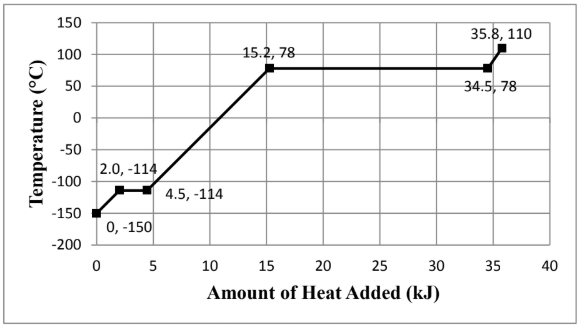# Problem: Consider the heating curve shown below for 22.86 grams of an unknown substance. You may assume constant pressure conditions. Note: the points are labeled as (amount of heat added, temperature). Calculate the amount of heat required (in kJ) to vaporize the compound whose heating curve is shown above. Show all work and circle your final answer.

###### FREE Expert Solution

We are asked to calculate the amount of heat to vaporize 22.86 grams of an unknown substance given the heating curve.

Phase changes occur on the horizontal parts of the curve since there are no temperature changes.###### Problem Details

Consider the heating curve shown below for 22.86 grams of an unknown substance. You may assume constant pressure conditions. Note: the points are labeled as (amount of heat added, temperature).Calculate the amount of heat required (in kJ) to vaporize the compound whose heating curve is shown above. Show all work and circle your final answer.